Matching Arrays
Matching Equal Groups
Drawing Arrays
Drawing Equal Groups
100

What multiplication problem does this array represent?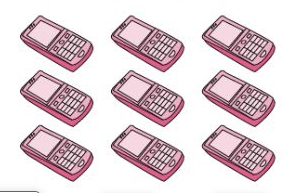3 x 3 = 9

100

What multiplication equation does these equal groups represent?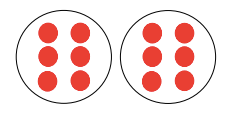2 x 6  = 12

100

Draw an array to solve 4 x 3.

You will have to show me in the camera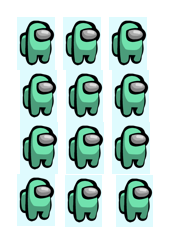100

Draw equal groups to solve 3 x 2.

You will have to show me in the camera100

What is the multiplication equation for

2+2+2 =

3 x 2 = 6

200

What multiplication equation does this array represent?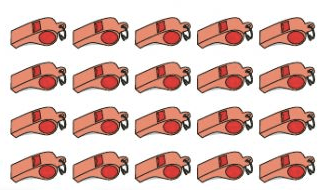4 x 5 = 20

200

What multiplication equation does these equal groups represent?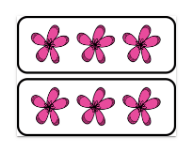2 x 3 = 6

200

Draw an array to solve 3 x 6.

You will have to show me in the camera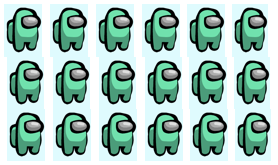200

Draw equal groups to solve 3 x 5.

You will have to show me in the camera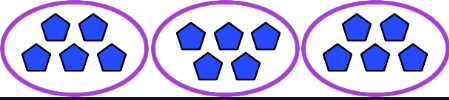200

What is the multiplication equation for

3+3+3+3+3+3=

6x3=

300

What multiplication equation does this array represent?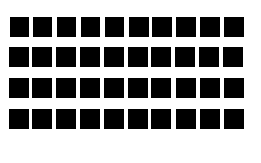4 x 10 = 40

300

What multiplication equation does these equal groups represent?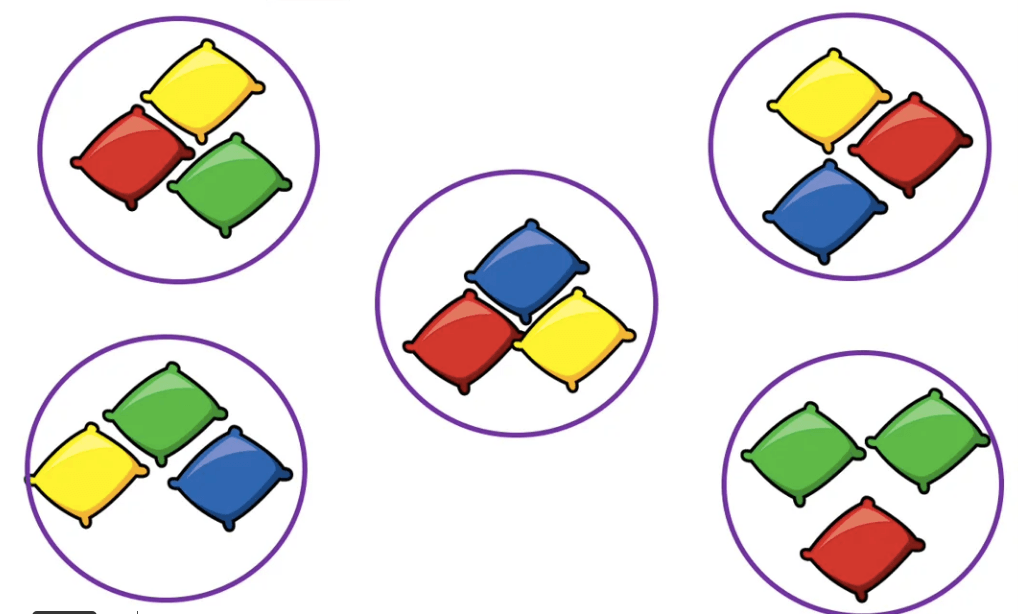5 x 3 = 15

300

Draw an array to solve 5 x 4.

You will have to show me in the camera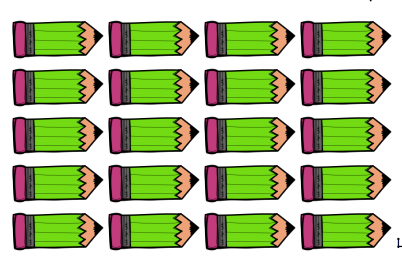300

Draw equal groups to solve 7 x 3.

You will have to show me in the camera

300

What is the multiplication equation for

16 + 16+ 16 + 16 + 16=

5x16=

400

What multiplication equation does this array represent?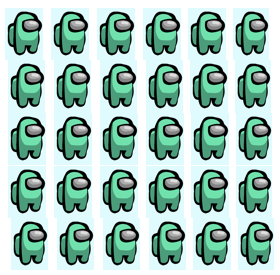5 x 6 = 30

400

What multiplication equation does these equal groups represent?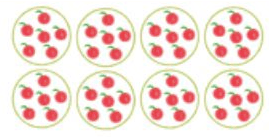8 x 6 = 48

400

Draw an array to solve 5 x 6.

You will have to show me in the camera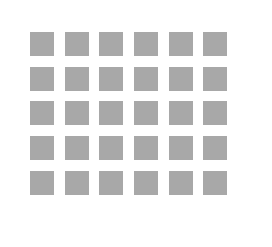400

Draw equal groups to solve 9 x 3.

You will have to show me in the camera

400

132+132 =

2x132=

500

What multiplication equation does this array represent?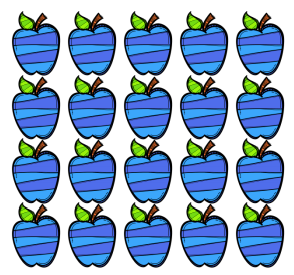4 x 5 = 20

500

What multiplication equation does these equal groups represent?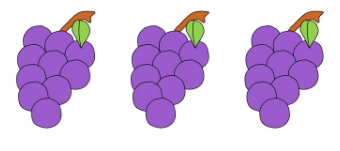3 x 10 = 30

500

Draw an array to solve 4 x 10.

You will have to show me in the camera500

Draw equal groups to solve 7 x 10.

You will have to show me in the camera

500

What is the multiplication equation for

17+ 17+ 17+ 17+ 17+ 17+ 17+ 17 + 17 + 17 + 17 =

12x11=

Click to zoom